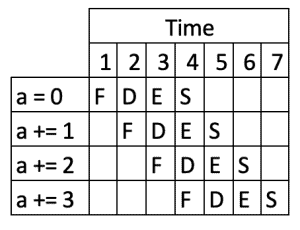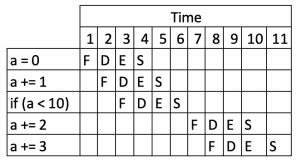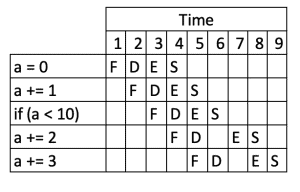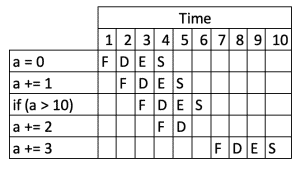## 1. Introduction

Branch Prediction is an interesting concept in computer science and can have a profound impact on the performance of our applications. Yet it’s generally not well understood and most developers pay very little attention to it.

In this article, we are going to explore exactly what it is, how it affects our software, and what we can do about it.

## 2. What Are Instruction Pipelines?

When we write any computer program, we are writing a set of commands that we expect the computer to execute in sequence.

Early computers would run these one at a time. This means that each command gets loaded into memory, executed in its entirety, and only when it’s completed will the next one get loaded.

Instruction Pipelines are an improvement over this. They allow the processor to split the work into pieces and then perform different parts in parallel. This would then allow the processor to execute one command while loading the next, ready to go.

Longer pipelines inside the processor not only allow each part to be simplified but also allow more parts of it to be performed in parallel. This can improve the overall performance of the system.

For example, we could have a simple program:

``````int a = 0;
a += 1;
a += 2;
a += 3;``````

This might be processed by a pipeline comprising of Fetch, Decode, Execute, Store segments as:We can see here how the overall execution of the four commands is run in parallel, thus making the entire sequence faster.

## 3. What Are the Hazards?

Certain the commands that the processor needs to execute will cause problems for the pipelining. These are any commands where the execution of one part of the pipeline is dependent on earlier parts, but where those earlier parts might not yet have been executed.

Branches are a specific form of hazard. They cause the execution to go in one of two directions, and it isn’t possible to know which direction until the branch is resolved. This means that any attempt to load the commands past the branch isn’t safe because we have no way of knowing where to load them from.

Let’s change our simple program to introduce a branch:

``````int a = 0;
a += 1;
if (a < 10) {
a += 2;
}
a += 3;``````

The result of this is the same as before, but we’ve introduced an if statement in the middle of it. The computer will see this and won’t be able to load commands past this until it’s been resolved. As such, the flow will look something like:We can immediately see the impact that this has on the execution of our program, and how many clock steps it took to execute the same result.

## 4. What Is Branch Prediction?

Branch Prediction is an enhancement to the above, where our computer will attempt to predict which way a branch is going to go and then act accordingly.

In our above example, the processor might predict that if (a < 10) is likely to be true, and so it will act as if the instruction a += 2 was the next one to execute. This would then cause the flow to look something like:We can see straight away that this has improved the performance of our program – it’s now taking nine ticks and not 11, so it’s 19% faster.

This is not without risk, though. If the branch prediction gets it wrong, then it will start to queue up instructions that shouldn’t be performed. If this happens, then the computer will need to throw them away and start over.

Let’s turn our conditional around so that it’s now false:

``````int a = 0;
a += 1;
if (a > 10) {
a += 2;
}
a += 3;``````

This might execute something like:This is now slower than the earlier flow, even though we’re doing less! The processor incorrectly predicted that the branch would evaluate to true, started queueing up the a += 2 instruction, and then had to discard it and start over when the branch evaluated to false.

## 5. Real Impact on Code

Now that we know what branch prediction is and what the benefits are, how can it affect us? After all, we’re talking about losing a few processor cycles on high-speed computers, so surely it won’t be noticeable.

And sometimes that’s true. But sometimes it can make a surprising difference to the performance of our applications. It depends a lot on exactly what we’re doing. Specifically, it depends on how much we’re doing in a short time.

### 5.1. Counting List Entries

Let’s try counting entries in a list. We’re going to generate a list of numbers, then count how many of them are less than a certain cutoff. That’s very similar to the above examples, but we’re doing it in a loop instead of just as a single instruction:

``````List<Long> numbers = LongStream.range(0, top)
.boxed()
.collect(Collectors.toList());

if (shuffle) {
Collections.shuffle(numbers);
}

long cutoff = top / 2;
long count = 0;

long start = System.currentTimeMillis();
for (Long number : numbers) {
if (number < cutoff) {
++count;
}
}
long end = System.currentTimeMillis();

LOG.info("Counted {}/{} {} numbers in {}ms",
count, top, shuffle ? "shuffled" : "sorted", end - start);``````

Note that we’re only timing the loop that does the counting because this is what we’re interested in. So, how long does this take?

If we’re generating sufficiently small lists, then the code runs so fast that it can’t be timed — a list of size 100,000 still shows a time of 0ms. However, when the list gets large enough that we can time it, we can see a significant difference based on whether we have shuffled the list or not. For a list of 10,000,000 numbers:

• Sorted – 44ms
• Shuffled – 221ms

That is, the shuffled list takes 5x longer to count than the sorted list, even though the actual numbers being counted are the same.

However, the act of sorting the list is significantly more expensive than just performing the counting. We should always profile our code and determine if any performance gains are beneficial.

### 5.2. Order of Branches

Following the above, it seems reasonable that the order of branches in an if/else statement should be important. That is, we could expect the following to perform better than if we re-ordered the branches:

``````if (mostLikely) {
// Do something
} else if (lessLikely) {
// Do something
} else if (leastLikely) {
// Do something
}``````

However, modern computers can avoid this issue by using the branch prediction cache. Indeed, we can test this as well:

``````List<Long> numbers = LongStream.range(0, top)
.boxed()
.collect(Collectors.toList());
if (shuffle) {
Collections.shuffle(numbers);
}

long cutoff = (long)(top * cutoffPercentage);
long low = 0;
long high = 0;

long start = System.currentTimeMillis();
for (Long number : numbers) {
if (number < cutoff) {
++low;
} else {
++high;
}
}
long end = System.currentTimeMillis();

LOG.info("Counted {}/{} numbers in {}ms", low, high, end - start);``````

This code executes in around the same time – ~35ms for sorted numbers, ~200ms for shuffled numbers – when counting 10,000,000 numbers, irrespective of the value of cutoffPercentage.

This is because the branch predictor is handling both branches equally and correctly guessing which way we’re going to go for them.

### 5.3. Combining Conditions

What if we have a choice between one or two conditions? It might be possible to re-write our logic in a different way that has the same behavior, but should we do this?

As an example, if we are comparing two numbers to 0, an alternative approach is to multiply them together and compare the result to 0. This is then replacing a condition with a multiplication. But is this worthwhile?

Let’s consider an example:

``````long[] first = LongStream.range(0, TOP)
.map(n -> Math.random() < FRACTION ? 0 : n)
.toArray();
long[] second = LongStream.range(0, TOP)
.map(n -> Math.random() < FRACTION ? 0 : n)
.toArray();

long count = 0;
long start = System.currentTimeMillis();
for (int i = 0; i < TOP; i++) {
if (first[i] != 0 && second[i] != 0) {
++count;
}
}
long end = System.currentTimeMillis();

LOG.info("Counted {}/{} numbers using separate mode in {}ms", count, TOP, end - start);``````

Our condition inside the loop can be replaced, as described above. Doing so actually does affect the runtime:

• Separate conditions – 40ms
• Multiple and single condition – 22ms

So the option that uses two different conditions actually takes twice as long to execute.

## 6. Conclusion

We’ve seen what branch prediction is and how it can have an impact on our programs. This can give us some additional tools in our belt to ensure that our programs are as efficient as possible.

However, as is always the case, we need to remember to profile our code before making major changes. It can sometimes be the case that making changes to help branch prediction costs more in other ways.

Examples of the cases from this article are available over on GitHub.

### Get started with Spring 5 and Spring Boot 2, through the Learn Spring course:

>> CHECK OUT THE COURSE